# Distance Time Graph Time Distance Time Graph Distance

• Slides: 16
Download presentation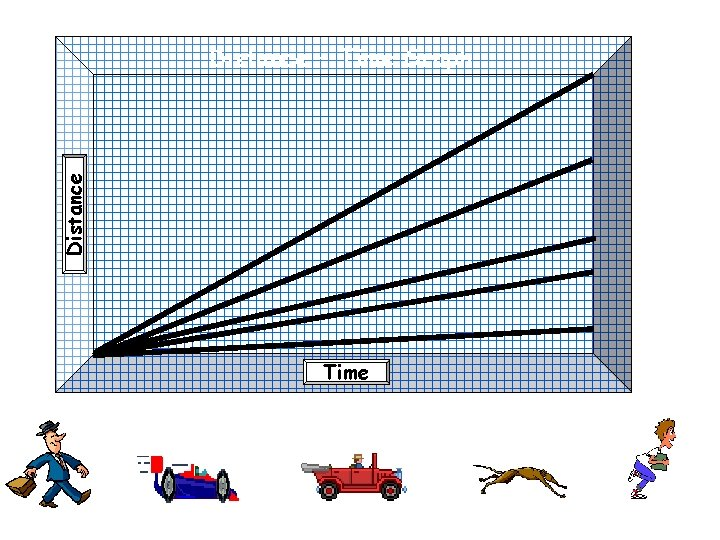Distance – Time Graph Time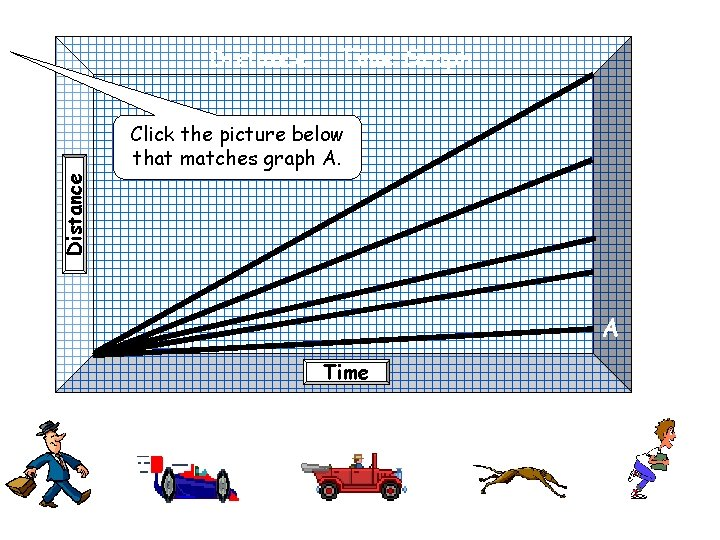Distance – Time Graph Distance Click the picture below that matches graph A. A TimeDistance – Time Graph Distance Click the picture below that matches graph B. B A Time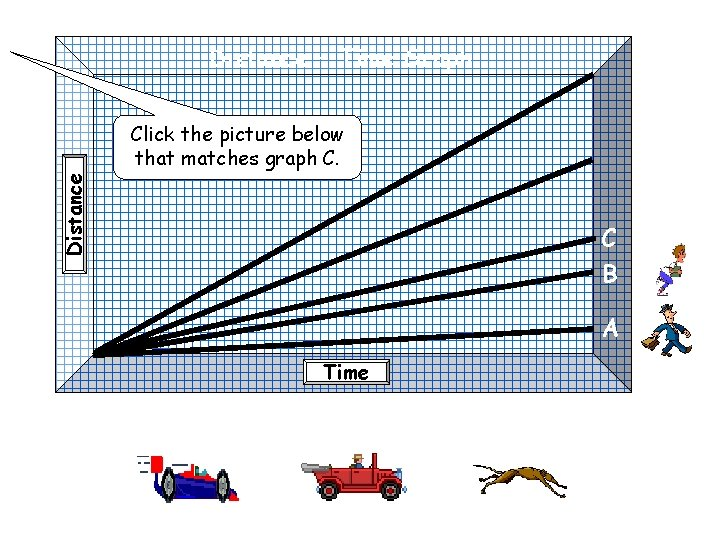Distance – Time Graph Distance Click the picture below that matches graph C. C B A Time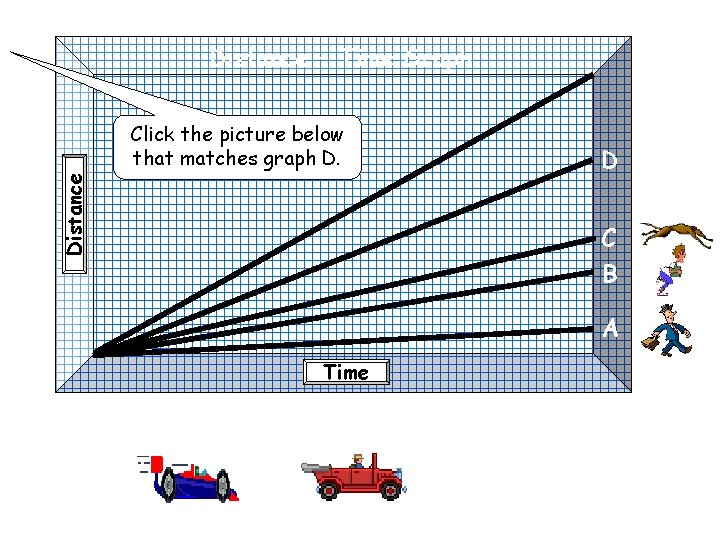Distance – Time Graph Distance Click the picture below that matches graph D. D C B A TimeDistance – Time Graph Distance Click the picture below that matches graph E. E D C B A Time Steeper means faster!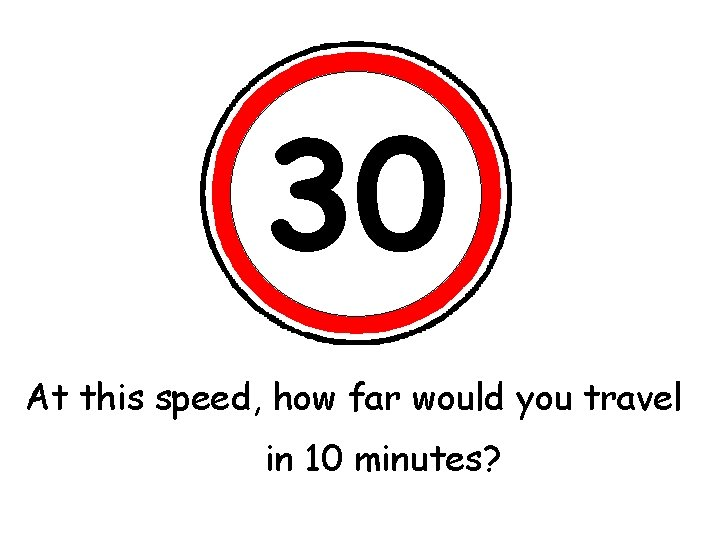30 At this speed, would you travel What’show the far speed limit? 2 hours? in ½ an hour? 10 minutes? 1 hour?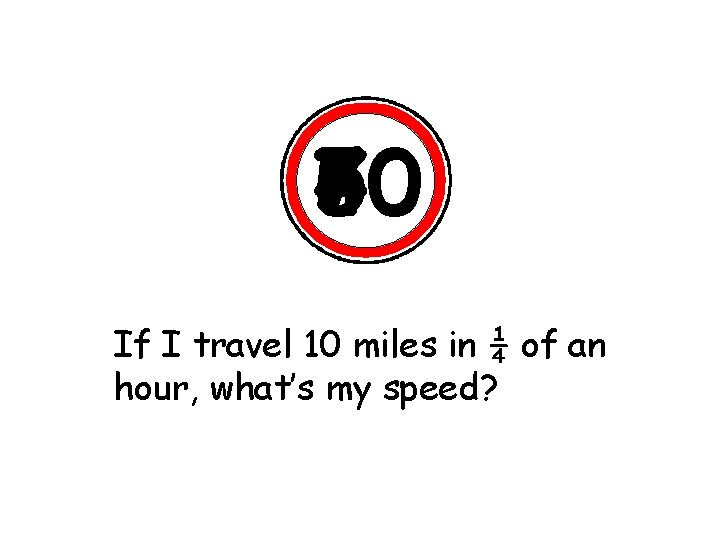60 70 50 40 If II travel 25 10 of 15 miles in ¼ If 70 1 hour, ½ an an hour, what’s my speed? what’s hour, my speed? my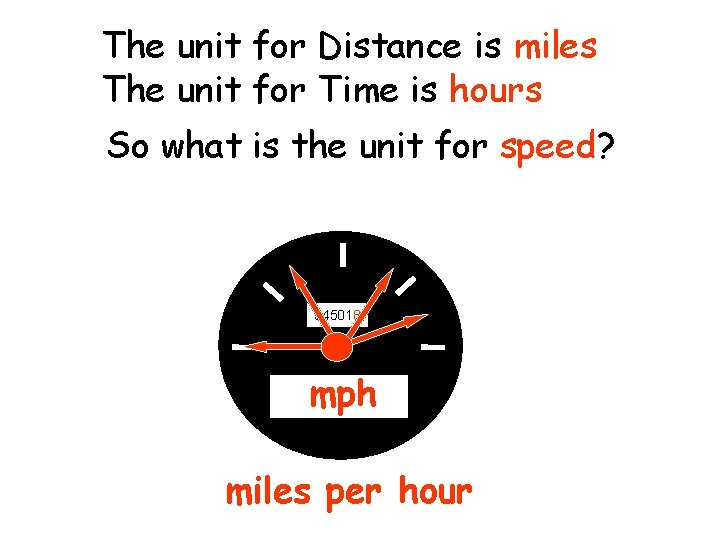The unit for Distance is miles The unit for Time is hours So what is the unit for speed? 345018 mph miles per hour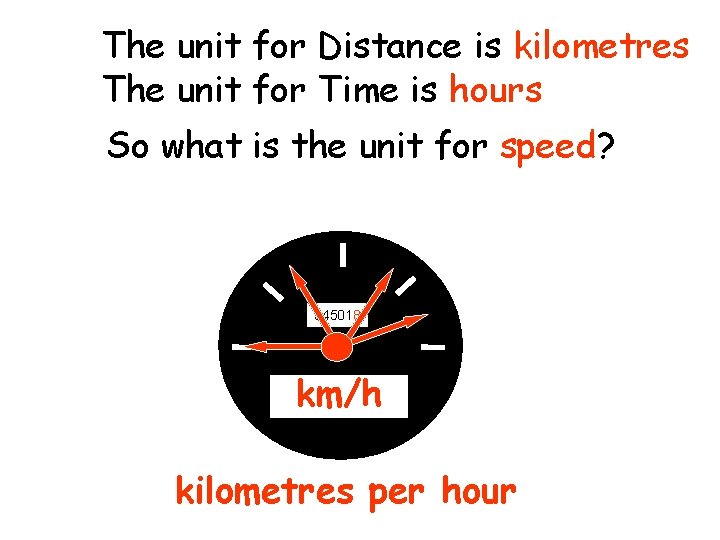The unit for Distance is kilometres The unit for Time is hours So what is the unit for speed? 345018 km/h kilometres per hour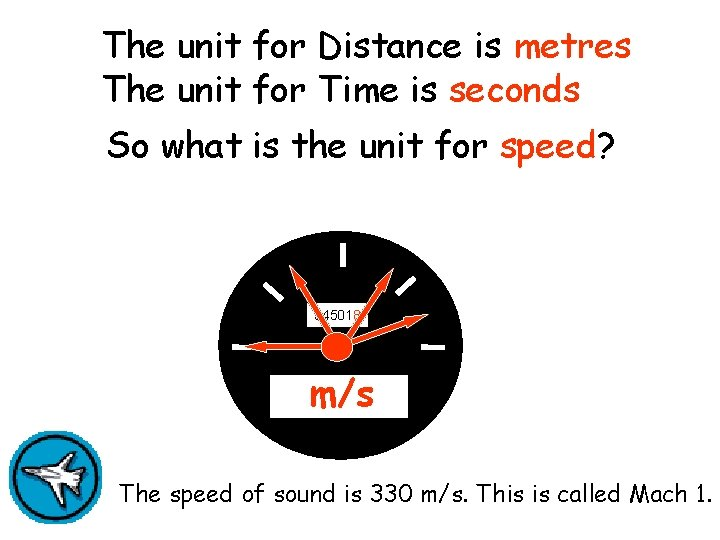The unit for Distance is metres The unit for Time is seconds So what is the unit for speed? 345018 m/s The speed of sound is 330 m/s. This is called Mach 1. metres per second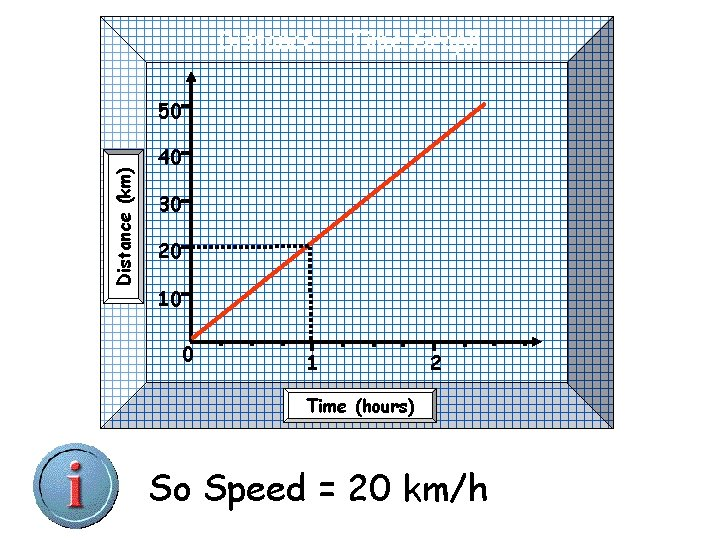Distance – Time Graph Distance (km) 50 40 30 20 10 0 1 2 Time (hours) What speed? hour to= travel 20 km So 1 Speed 20 km/hDistance – Time Graph Distance (miles) 50 40 30 20 10 0 ? 1 2 Time (hours) What speed? 2 So hours to =travel 30 miles Speed 15 mphNow do Exercise 1, Q. 1, 2 & 3 (HSDU Support Materials)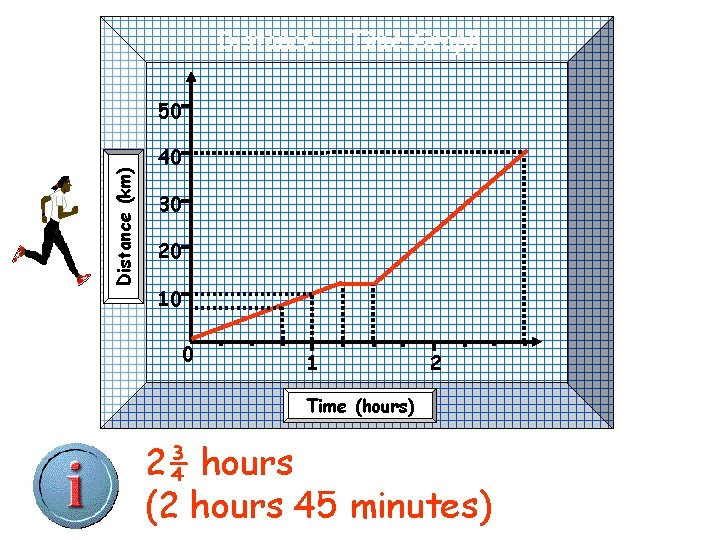Distance – Time Graph Distance (km) 50 40 30 20 10 0 1 2 Time (hours) 10 km inhour 1 in hour, so 10 km/h. 2¾ hours ¼ of an What was the speed for the How long did take to run she stop for? Approximately 7½ kilometres. How far ¾ it of an hour? (2 (15 minutes) first part 45 ofminutes) the journey? 40 hours km?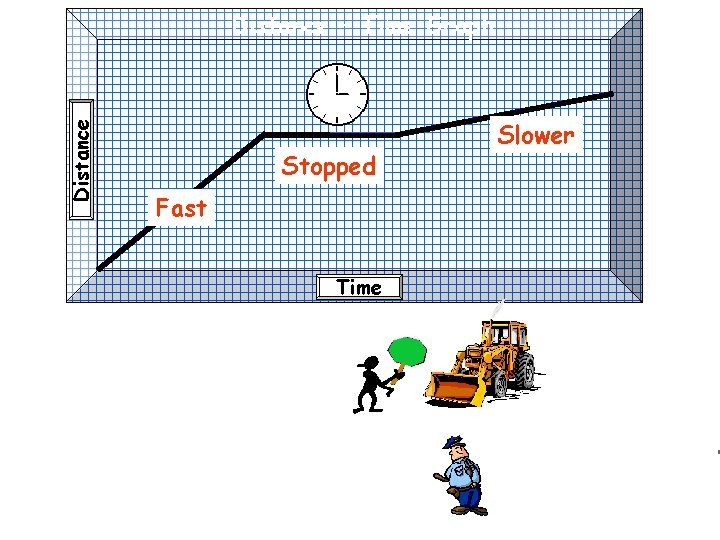Distance – Time Graph Stopped Fast Time Slower